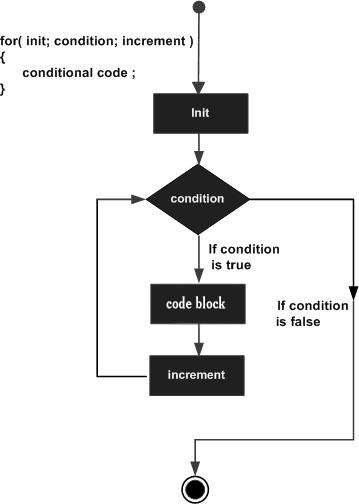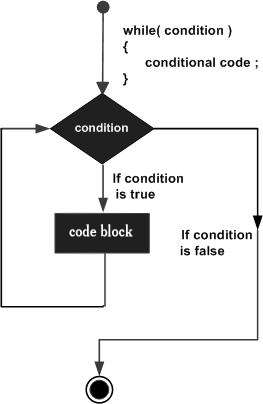# Difference Between for and while loop

In this post, we will understand the difference between the ‘for’ and the ‘while’ loop.

## For loop

• The initialization, condition checking, and the iteration statements are written at the beginning of the loop.

• It is used only when the number of iterations is known beforehand.

• If the condition is not mentioned in the 'for' loop, then the loop iterates infinite number of times.

• The initialization is done only once, and it is never repeated.

• The iteration statement is written at the beginning.

• Hence, it executes once all statements in loop have been executed.

## Example

for(initialization; condition; iteration){
//body of the 'for' loop
}

Following is the flowchart of for loop −## While condition

• The initialization and the condition checking is done at the beginning of the loop.

• It is used only when the number of iterations isn’t known.

• If the condition is not mentioned in the 'while' loop, it results in a compilation error.

• If the initialization is done when the condition is being checked, then initialization occurs every time the loop is iterated through.

• The iteration statement can be written within any point inside the loop.

## Example

while ( condition) {
statements;
//body of the loop
}

Following is the flowchart of while loop −